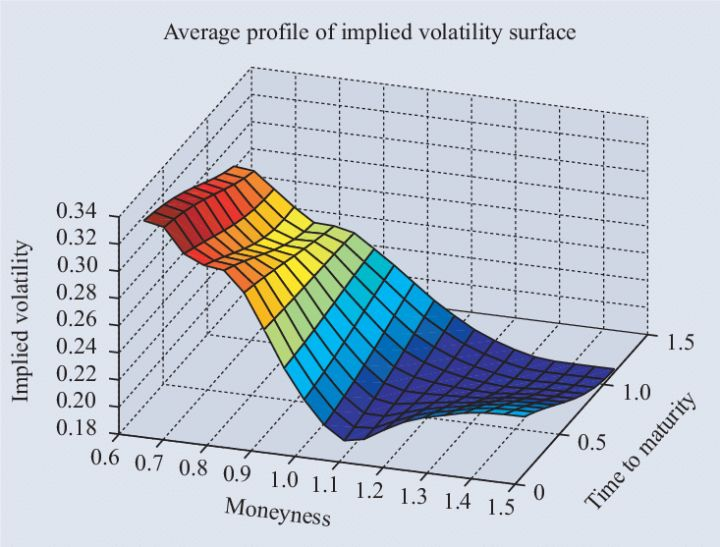# Introduction to Gamma Exposure(GEX)

Joey
May 21, 2022In past blogs we have introduced option greeks, where we define Gamma as change of rate on Delta given unit change of option underlying asset price. Gamma describes how fast the option value changes when the stock price changes a unit. In practice Gamma is a “gold mine” that often helps us effectively excavate trading opportunities. This is because Gamma plays a significant role in building a successful delta neutralization strategy and dynamic hedging. Gamma is also a core indicator that market makers would constantly monitor on. As the main opponent of mass traders, market makers has a huge demand over dynamic hedging to maintain their delta neutral condition and avoid directional risk as possible to play their bid and ask games. Market makers are often described as “giants in the market” as they influence the liquidity of the market and bid-ask spread using their informational and capital edge. However, on the flip side it is hard to totally hide a giant’s footstep in the market as their movement has momentum impact. Therefore, we as normal traders and investors with relatively small money pool can trace the footstep of giants and deduce their intention. If we can effectively analyse possible expected action from market makers and their footsteps, we would own a unique edge in the market. GEX indicator is exactly such a tool that can help us monitor the footsteps of market makers.

# Option Learning: Implied Volatility

Joey
April 13, 2022Volatility in option trading can be mainly categorized into historical volatility, implied volatility and future volatility. Historical volatility measures stock price fluctuation in selected historical interval, usually calculated as annualized standard deviation of price change. Implied volatility is a theoretical value as a calculated parameter assuming the option market price is approximately equal to the theoretical value of Black-Scholes pricing model. Future volatility in contrast to historical volatility is expected price change level in the future.

# Introduction on Greeks——Delta

Joey
April 13, 2022Fundamentally delta calculates the coupling coefficient and the price sensitivity of an option to its underlying asset. A long call option with delta value of 0.6 means that the option value would rise 0.6 dollar per stock when the underlying equity rises 1 dollar per unit. As the price change of an option would not surpass the price change of its underlying asset, delta is always valued between -1 and 1.

# Unusual Option Activity - Trace the footstep of WallStreet "smart money"